• 针对非正面姿态下人脸识别率低下的问题,提出一种基于双路循环生成对抗网络的多姿态人脸识别方法。该网络由人脸转正及人脸旋转两部分组成。人脸转正部分完成侧面人脸向正面人脸的转化,实现多对一的姿态类别映射;人脸...
• 在无人车夜视红外视频彩色化问题中, 考虑到可同时利用单帧图像的信息和视频的帧间信息, 提出了一种双通道循环生成对抗网络（DcCCAN）对夜视红外视频进行彩色化。DcCCAN是在循环一致生成对抗网络（CCAN）的基础上提出...
• 行业分类-物理装置-一种基于循环生成对抗网络的水下图像复原方法.zip
• 循环生成对抗网络CycleGan dataset https://people.eecs.berkeley.edu/~taesung_park/CycleGAN/datasets/vangogh2photo.zip GitHub地址：...
循环生成对抗网络CycleGan实现风格迁移
dataset
https://people.eecs.berkeley.edu/~taesung_park/CycleGAN/datasets/vangogh2photo.zip
GitHub地址：https://github.com/yunlong-G/tensorflow_learn/blob/master/GAN/CycleGan.ipynb environment
python=3.6tensorflow=1.13.1scipy=1.2.1keras=2.2.4keras-contrib=2.0.8
结构简介
如果要用普通GAN将照片转换为绘画（或着反过来），需要使用成对的图像进行训练。而CycleGAN是一种特殊的GAN，无须使用成对图像进行训练，便可以将图像从一个领域 变换到另一个领域 。CycleGAN训练学习两种映射的生成网络。绝大多数GAN训练只一个生成网络，而CycleGAN会训练两个生成网络和两个判别网络。CycleGAN包含如下两个生成网络。
生成网络A：学习映射

G

:

X

→

Y

G:X\rightarrow Y

，其中

X

X

是源领域，

Y

Y

是目标领域。该映射接收源领域

A

A

的图像，将其转换成和目标领域

B

B

中的图像相似的图像。简单说来，该网络旨在学习能使

G

(

X

)

G(X)

和

Y

Y

相似的映射。生成网络B：学习映射

F

:

Y

→

X

F:Y\rightarrow X

，接收目标领域

B

B

的图像，将其转换成和源领域

A

A

中图像相似的图像。类似地，该网络旨在学习能使

F

(

G

(

X

)

)

F(G(X))

和

X

X

相似的映射。
两个网络的架构相同，但都单独训练。
CycleGAN包含如下两个判别网络。
判别网络A：判别网络A负责区分生成网络B生成的图像（用

F

(

Y

)

F(Y)

表示）和源领域A中的真实图像（表示为

X

X

）。判别网络B：判别网络B负责区分生成网络A生成的图像（用$G(X) 表 示 ） 和 目 标 领 域 B 中 的 真 实 图 像 （ 表 示 为 表示）和目标领域B中的真实图像（表示为 Y$）。
两个网络的架构相同，也需要单独训练。
[外链图片转存失败,源站可能有防盗链机制,建议将图片保存下来直接上传(img-s465uGrQ-1620893448088)(attachment:268fb040-17fc-47fd-aca7-33fd02e347b5.png)]
损失函数
总损失函数

L

(

G

,

F

,

D

x

,

D

y

)

=

L

G

A

N

(

G

,

D

Y

,

X

,

Y

)

+

L

G

A

N

(

F

,

D

X

,

Y

,

X

)

+

λ

L

c

y

c

(

G

,

F

)

L(G,F,D_x,D_y) = L_{GAN}(G,D_Y,X,Y)+L_{GAN}(F,D_X,Y,X)+\lambda L_{cyc}(G,F)

对抗损失
对抗损失是来自概率分布A或概率分布B的图像和生成网络生成的图像之间的损失。该网络涉及两个映射函数，都需要应用对抗损失。

L

G

A

N

(

G

,

D

Y

,

X

,

Y

)

=

E

y

p

d

a

t

a

(

y

)

[

l

o

g

D

Y

(

y

)

]

+

E

x

p

d

a

t

a

(

x

)

[

l

o

g

(

1

−

D

Y

(

G

(

x

)

)

]

L_{GAN}(G,D_Y,X,Y)=E_{y~p_{data}(y)}[logD_Y(y)]+E_{x~p_{data}(x)}[log(1-D_Y(G(x))]

CycleGAN包含两个生成器G和F，对应两个判别器DX和DY，下面以生成器G和判别器DY进行分析（F和DX的原理与之相同）：

G

G

输入

X

X

绘画，输出

G

(

X

)

G(X)

图片，使

D

Y

D_Y

判断

G

(

X

)

G(X)

与

Y

Y

越来越相似。

F

F

输入

Y

Y

绘画，输出

F

(

Y

)

F(Y)

图片，使

D

X

D_X

判断

F

(

Y

)

F(Y)

与

X

X

越来越相似。
循环一致损失
对抗性损失能够让生成器

G

G

和生成器

F

F

学习到域

Y

Y

和域

X

X

的分布，但是却没有保证从

X

X

得到

G

(

X

)

G(X)

时图像的内容不变，因为

G

(

X

)

G(X)

只需要符合域

Y

Y

分布即可，并没有对其施加约束，所以

X

X

到

G

(

X

)

G(X)

包含很多种可能的映射。
利用循环一致性损失来进行约束，即

X

X

通过

G

G

生成

G

(

X

)

G(X)

后，再通过

F

F

，生成

F

(

G

(

X

)

)

F(G(X))

并使其接近于X。

L

c

y

c

(

G

,

F

)

=

E

x

p

d

a

t

a

(

x

)

[

∣

∣

F

(

G

(

x

)

)

−

x

∣

∣

1

]

+

E

y

p

d

a

t

a

(

y

)

[

∣

∣

G

(

F

(

y

)

)

−

y

∣

∣

1

]

L_{cyc}(G,F)=E_{x~p_{data}(x)}[||F(G(x))-x||_1]+E_{y~p_{data}(y)}[||G(F(y))-y||_1]

构建CycleGAN
# 导入需要的包
import time
from glob import glob

import matplotlib.pyplot as plt
import numpy as np
import tensorflow as tf
from keras import Input, Model
from keras.callbacks import TensorBoard
from keras.layers import Conv2D, BatchNormalization, Activation, Add, Conv2DTranspose, \
from keras_contrib.layers import InstanceNormalization

Using TensorFlow backend.

残差块
def residual_block(x):
"""
Residual block
"""
res = Conv2D(filters=128, kernel_size=3, strides=1, padding="same")(x)
res = BatchNormalization(axis=3, momentum=0.9, epsilon=1e-5)(res)
res = Activation('relu')(res)

res = Conv2D(filters=128, kernel_size=3, strides=1, padding="same")(res)
res = BatchNormalization(axis=3, momentum=0.9, epsilon=1e-5)(res)


生成器
def build_generator():
"""
Create a generator network using the hyperparameter values defined below
"""
input_shape = (128, 128, 3)
residual_blocks = 6
input_layer = Input(shape=input_shape)

# First Convolution block
x = Conv2D(filters=32, kernel_size=7, strides=1, padding="same")(input_layer)
x = InstanceNormalization(axis=1)(x)
x = Activation("relu")(x)

# 2nd Convolution block
x = Conv2D(filters=64, kernel_size=3, strides=2, padding="same")(x)
x = InstanceNormalization(axis=1)(x)
x = Activation("relu")(x)

# 3rd Convolution block
x = Conv2D(filters=128, kernel_size=3, strides=2, padding="same")(x)
x = InstanceNormalization(axis=1)(x)
x = Activation("relu")(x)

# Residual blocks
for _ in range(residual_blocks):
x = residual_block(x)

# Upsampling blocks

# 1st Upsampling block
x = Conv2DTranspose(filters=64, kernel_size=3, strides=2, padding='same', use_bias=False)(x)
x = InstanceNormalization(axis=1)(x)
x = Activation("relu")(x)

# 2nd Upsampling block
x = Conv2DTranspose(filters=32, kernel_size=3, strides=2, padding='same', use_bias=False)(x)
x = InstanceNormalization(axis=1)(x)
x = Activation("relu")(x)

# Last Convolution layer
x = Conv2D(filters=3, kernel_size=7, strides=1, padding="same")(x)
output = Activation('tanh')(x)

model = Model(inputs=[input_layer], outputs=[output])
return model

判别器
def build_discriminator():
"""
Create a discriminator network using the hyperparameter values defined below
"""
input_shape = (128, 128, 3)
hidden_layers = 3

input_layer = Input(shape=input_shape)

# 1st Convolutional block
x = Conv2D(filters=64, kernel_size=4, strides=2, padding="valid")(x)
x = LeakyReLU(alpha=0.2)(x)

# 3 Hidden Convolution blocks
for i in range(1, hidden_layers + 1):
x = Conv2D(filters=2 ** i * 64, kernel_size=4, strides=2, padding="valid")(x)
x = InstanceNormalization(axis=1)(x)
x = LeakyReLU(alpha=0.2)(x)

# Last Convolution layer
output = Conv2D(filters=1, kernel_size=4, strides=1, activation="sigmoid")(x)

model = Model(inputs=[input_layer], outputs=[output])
return model

载入图片
def load_images(data_dir):
imagesA = glob(data_dir + '/testA/*.*')
imagesB = glob(data_dir + '/testB/*.*')

allImagesA = []
allImagesB = []

for index, filename in enumerate(imagesA):

imgA = imresize(imgA, (128, 128))
imgB = imresize(imgB, (128, 128))

if np.random.random() > 0.5:
imgA = np.fliplr(imgA)
imgB = np.fliplr(imgB)

allImagesA.append(imgA)
allImagesB.append(imgB)

# Normalize images
allImagesA = np.array(allImagesA) / 127.5 - 1.
allImagesB = np.array(allImagesB) / 127.5 - 1.

return allImagesA, allImagesB

imagesA = glob(data_dir + '/testA/*.*')
imagesB = glob(data_dir + '/testB/*.*')

imagesA = np.random.choice(imagesA, batch_size)
imagesB = np.random.choice(imagesB, batch_size)

allA = []
allB = []

for i in range(len(imagesA)):
# Load images and resize images
imgA = imresize(imread(imagesA[i], mode='RGB').astype(np.float32), (128, 128))
imgB = imresize(imread(imagesB[i], mode='RGB').astype(np.float32), (128, 128))

allA.append(imgA)
allB.append(imgB)

return np.array(allA) / 127.5 - 1.0, np.array(allB) / 127.5 - 1.0


保存训练结果
def save_images(originalA, generatedB, recosntructedA, originalB, generatedA, reconstructedB, path):
"""
Save images
"""
fig = plt.figure()
ax.imshow(originalA)
ax.axis("off")
ax.set_title("Original")

ax.imshow(generatedB)
ax.axis("off")
ax.set_title("Generated")

ax.imshow(recosntructedA)
ax.axis("off")
ax.set_title("Reconstructed")

ax.imshow(originalB)
ax.axis("off")
ax.set_title("Original")

ax.imshow(generatedA)
ax.axis("off")
ax.set_title("Generated")

ax.imshow(reconstructedB)
ax.axis("off")
ax.set_title("Reconstructed")

plt.savefig(path)

def write_log(callback, name, loss, batch_no):
"""
Write training summary to TensorBoard
"""
summary = tf.Summary()
summary_value.simple_value = loss
summary_value.tag = name
callback.writer.flush()

集成训练
if __name__ == '__main__':
data_dir = "../dataset/vangogh2photo/"
batch_size = 4
epochs = 1000
mode = 'train'

if mode == 'train':
# 载入数据

# 迭代器设置

#定义网络，训练判别器
discriminatorA = build_discriminator()
discriminatorB = build_discriminator()

discriminatorA.compile(loss='mse', optimizer=common_optimizer, metrics=['accuracy'])
discriminatorB.compile(loss='mse', optimizer=common_optimizer, metrics=['accuracy'])

# 构建生成网络
generatorAToB = build_generator()
generatorBToA = build_generator()

"""
创建对抗网络
"""
inputA = Input(shape=(128, 128, 3))
inputB = Input(shape=(128, 128, 3))

# 构建两个生成器
generatedB = generatorAToB(inputA)
generatedA = generatorBToA(inputB)

# 构建重构网络
reconstructedA = generatorBToA(generatedB)
reconstructedB = generatorAToB(generatedA)

generatedAId = generatorBToA(inputA)
generatedBId = generatorAToB(inputB)

# 使判别器不被训练
discriminatorA.trainable = False
discriminatorB.trainable = False

probsA = discriminatorA(generatedA)
probsB = discriminatorB(generatedB)

# 整体网络构建
outputs=[probsA, probsB, reconstructedA, reconstructedB,
generatedAId, generatedBId])
adversarial_model.compile(loss=['mse', 'mse', 'mae', 'mae', 'mae', 'mae'],
loss_weights=[1, 1, 10.0, 10.0, 1.0, 1.0],
optimizer=common_optimizer)
# 利用tensorboard记录训练数据
write_graph=True)
tensorboard.set_model(generatorAToB)
tensorboard.set_model(generatorBToA)
tensorboard.set_model(discriminatorA)
tensorboard.set_model(discriminatorB)

real_labels = np.ones((batch_size, 7, 7, 1))
fake_labels = np.zeros((batch_size, 7, 7, 1))

for epoch in range(epochs):
print("Epoch:{}".format(epoch))

dis_losses = []
gen_losses = []

num_batches = int(min(imagesA.shape, imagesB.shape) / batch_size)
print("Number of batches:{}".format(num_batches))

for index in range(num_batches):
print("Batch:{}".format(index))

# 获得样例图片
batchA = imagesA[index * batch_size:(index + 1) * batch_size]
batchB = imagesB[index * batch_size:(index + 1) * batch_size]

# 利用生成器生成图片
generatedB = generatorAToB.predict(batchA)
generatedA = generatorBToA.predict(batchB)

# 对判别器A训练区分真假图片
dALoss1 = discriminatorA.train_on_batch(batchA, real_labels)
dALoss2 = discriminatorA.train_on_batch(generatedA, fake_labels)

# 对判别器B训练区分真假图片
dBLoss1 = discriminatorB.train_on_batch(batchB, real_labels)
dbLoss2 = discriminatorB.train_on_batch(generatedB, fake_labels)

# 计算总的loss值

print("d_loss:{}".format(d_loss))

"""
训练生成网络
"""
[real_labels, real_labels, batchA, batchB, batchA, batchB])

print("g_loss:{}".format(g_loss))

dis_losses.append(d_loss)
gen_losses.append(g_loss)

"""
每一次都保留loss值
"""
write_log(tensorboard, 'discriminator_loss', np.mean(dis_losses), epoch)
write_log(tensorboard, 'generator_loss', np.mean(gen_losses), epoch)

# 每10个世代进行一次效果展示
if epoch % 10 == 0:
# 得到测试机图片A,B

# 利用生成器生成图片
generatedB = generatorAToB.predict(batchA)
generatedA = generatorBToA.predict(batchB)

# 的到重构图片
reconsA = generatorBToA.predict(generatedB)
reconsB = generatorAToB.predict(generatedA)

# 保存原生图片，生成图片，重构图片
for i in range(len(generatedA)):
save_images(originalA=batchA[i], generatedB=generatedB[i], recosntructedA=reconsA[i],
originalB=batchB[i], generatedA=generatedA[i], reconstructedB=reconsB[i],
path="results/gen_{}_{}".format(epoch, i))

# 保存模型
generatorAToB.save_weights("./weight/generatorAToB.h5")
generatorBToA.save_weights("./weight/generatorBToA.h5")
discriminatorA.save_weights("./weight/discriminatorA.h5")
discriminatorB.save_weights("./weight/discriminatorB.h5")

elif mode == 'predict':
# 构建生成网络并载入权重
generatorAToB = build_generator()
generatorBToA = build_generator()

# 获得预测的图片

# 保存预测图片
generatedB = generatorAToB.predict(batchA)
generatedA = generatorBToA.predict(batchB)

reconsA = generatorBToA.predict(generatedB)
reconsB = generatorAToB.predict(generatedA)

for i in range(len(generatedA)):
save_images(originalA=batchA[i], generatedB=generatedB[i], recosntructedA=reconsA[i],
originalB=batchB[i], generatedA=generatedA[i], reconstructedB=reconsB[i],
path="results/test_{}".format(i))


训练30个世代训练50个世代训练100世代预测结果
只需要将main中mode = 'train’改成mode = ‘predict’，再提一下就是，main中的epoch = 1000 大家更具自己需要重新设置就好，还有现在的代码是都训练完才会保存模型，可以修改成10个世代保存一次。训练过程记录
loss值
我们在训练时利用tensorboard记录的判别器和生成器的loss值，之后可以在cmd窗口到logs目录前，使用tensorboard --logdir = ./logs命令可视化训练过程loss值变化同时，通过上部的选项按键可以看到网络的结构图小结
本次实验利用CycleGan实现油画风格到照片风格的转变，因为利用自己电脑运行代码，只运行了100个世代已经花费了两个多小时，达到的按效果还不是很明显，可以看到的是图片转成油画比较接近了，但是优化到照片还缺少了一定的清晰度。在之后的学习再尝试别的的网络学习了。
展开全文python 深度学习
• 原文： https://blog.csdn.net/qq_21190081/article/details/78807931 https://www.jianshu.com/p/64bf39804c80 1. 单向 GAN ...2. 循环 GAN 而循环对抗生成网络可以看作两个普通的GAN...
原文：
https://blog.csdn.net/qq_21190081/article/details/78807931https://www.jianshu.com/p/64bf39804c80
1. 单向 GAN
https://blog.csdn.net/nymph_h/article/details/96432920
2. 循环 GAN
而循环对抗生成网络可以看作两个普通的GAN分别首位相接，如图所示。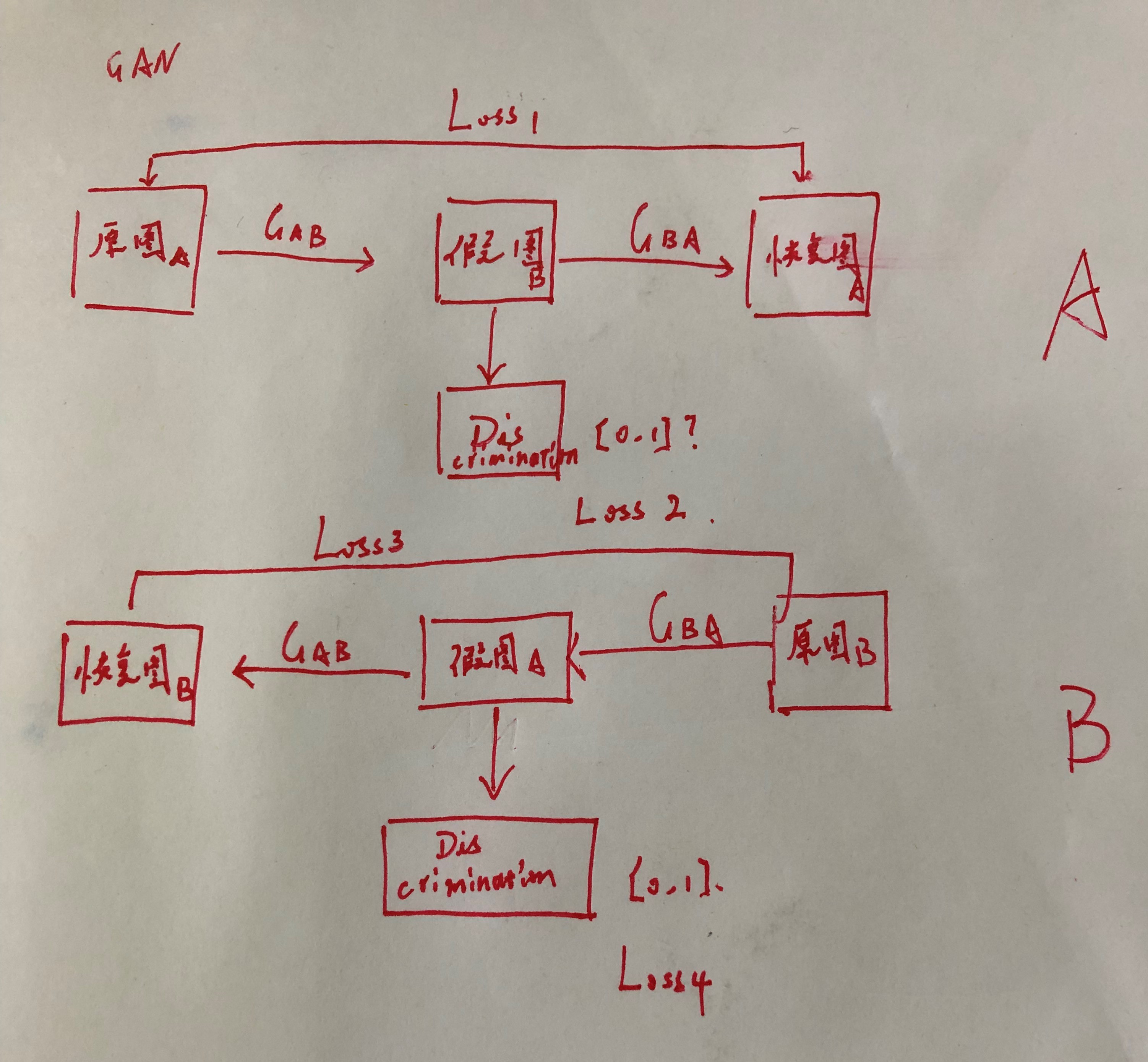看上去就是两个原本的GAN头尾叠在一起了，但是注意，相比原图，中间结果要求是服从另一个图片域的假图，而不是抽象的代码。下面是别人的图片。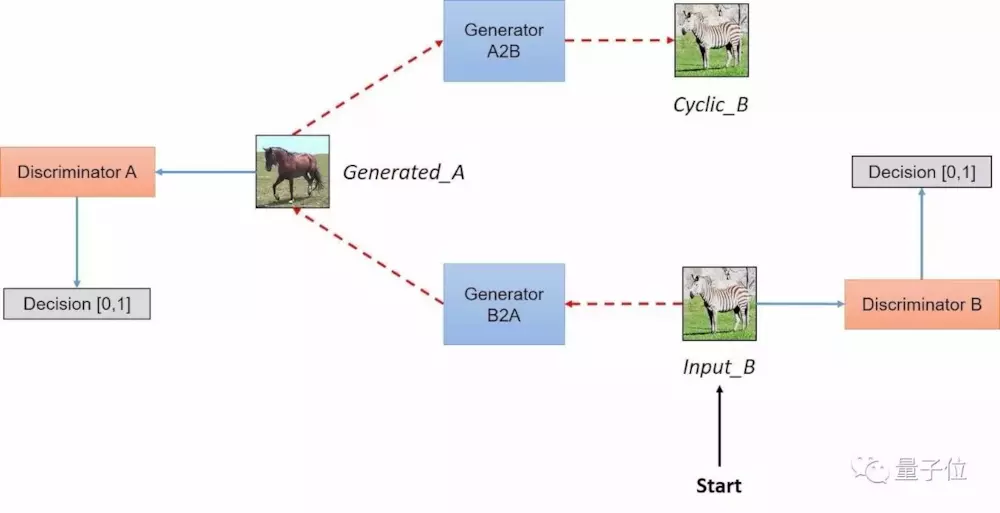与原来的普通GAN相比，损失函数由两个变为了四个（容易理解）。 程序的工作流程变为：
从域A选择一张图片input_A，通过Gab变换为域B中的一张假图片Gen_B，计算分类器Discrimination_B的损失将假图片通过Gba变换回域A，得到Cyclic_A，计算它与input_A之间的损失另一半网络，执行相同的步骤。
可以实现无配对的两个图片集的训练是CycleGAN与Pixel2Pixel相比的一个典型优点。但是我们仍然需要通过训练创建这个映射来确保输入图像和生成图像间存在有意义的关联，即输入输出共享一些特征。
展开全文• 本文综合整理常用的神经网络，包括生物神经网络、人工神经网络、卷积神经网络、循环神经网络、生成对抗网络；参考了许多高校的课程、论文、博客和视频等。文章的结构是先进行概念了解，然后结合图片、结构图、一步...
前言
本文综合整理常用的神经网络，包括生物神经网络、人工神经网络、卷积神经网络、循环神经网络、生成对抗网络；参考了许多高校的课程、论文、博客和视频等。文章的结构是先进行概念了解，然后结合图片、结构图、一步一步详细讲解；大家要不看看？ ( •̀ ω •́ )y

一、人工神经网络
简介：人工神经网络 (Artificial Neural Network, ANN)，由人工神经元构成的网络，模拟人类的大脑；它模拟生物过程以反映人脑某些特征的计算结构。
联系：人工神经元模拟生物神经元；人工神经网络模拟人类的大脑，模拟生物神经网络。
特点：人工神经网络是一个并行、分布处理结构，具有学习能力、泛化能力。
功能：联想记忆功能、非线性映射功能、分类与识别功能、知识处理功能。
详细介绍：一篇文章“简单”认识《人工神经网络》(更新版)
目录大纲：

展开全文人工智能 深度学习
• 生成对抗网络和对抗神经网络 如果您最近一直在关注人工智能（AI）新闻媒体，则可能听说过Google的顶级AI人物之一Ian Goodfellow于三月份移居了Apple 。 Goodfellow在2017年被麻省理工学院评为35岁以下创新者之一，在...

生成对抗网络和对抗神经网络
如果机器学习是我们应该学习的东西，而GAN是机器学习中最热门的想法，那么可以肯定的是，更多地了解GAN是个好主意。
互联网上有很多有趣的文章，视频和其他学习材料可以解释GAN。 本文是GAN和一些开源项目和资源的非常入门的指南，您可以在其中扩展您对GAN和机器学习的知识。
统计分类模型
在学习GAN之前，重要的是要了解广泛用于机器学习和统计的统计分类模型之间的差异。 在统计分类中，这是一个研究领域，旨在确定一组类别中新观察结果的位置，其中有两个主要模型： 判别模型和生成模型。 判别模型属于监督学习 ，生成模型属于非监督学习。 斯坦福大学演讲的这张幻灯片解释了两者的区别：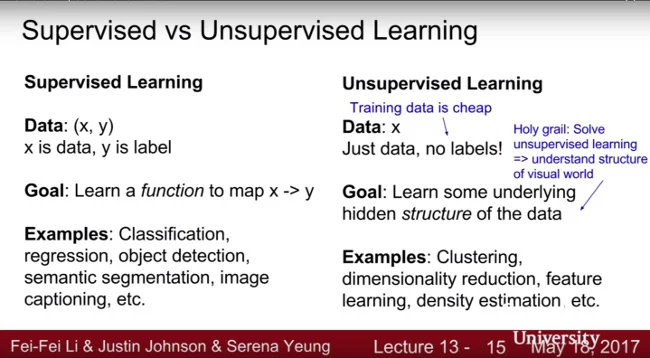判别模型
区分模型尝试根据从新项目中发现的特征来识别特定新项目在类别中的位置。 例如，假设我们要创建一个非常简单的区分模型系统，该系统将给定的对话分配给特定的电影类别，在这种情况下，是否来自电影《复仇者联盟》。 这是一行示例对话：

“他是复仇者联盟的一员，该组织将尽一切力量确保世界安全。Loki最好提防。”

我们预先定义的“复仇者联盟”关键字组可以包括“复仇者联盟”和“ Loki”。 我们的判别模型算法将尝试匹配给定文本中与“复仇者联盟”相关的关键字，并报告在0.0到1.0之间的概率，其中接近1的数字表示最有可能来自“复仇者联盟”的对话， 而接近0的数字表明事实并非如此。
这是判别模型的一个非常简单的示例，但是您可能会明白。 您可以看到，区分模型在很大程度上取决于预定义类别的质量，但是仅作了一些假设。
生成模型
生成模型不是通过标记数据而是通过尝试从特定数据集中获取一些见识来工作。 如果您还记得高中时可能学过的高斯分布，则可能马上就想到了。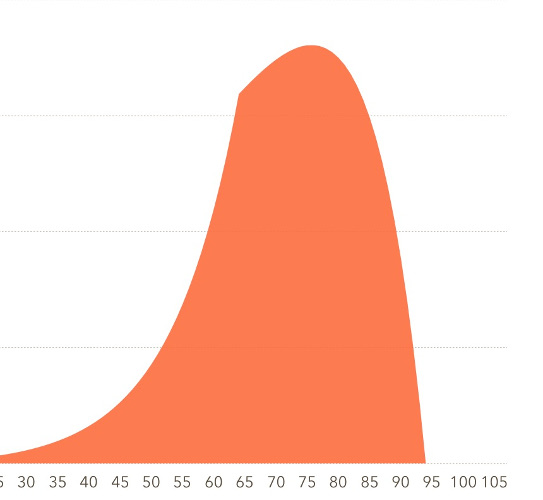例如，假设这是一班100名学生的期末考试成绩列表。

10名学生达到80％或以上  80名学生获得70％至80％  10名学生的分数达到或低于70％

根据这些数据，我们可以推断出大多数学生在70年代得分。 此洞察力基于特定数据集的统计分布。 如果我们看到其他模式，也可以收集该数据并进行其他推断。
您可能听说过的生成模型包括高斯混合模型，贝叶斯模型，隐马尔可夫模型和变异编码器模型。 GAN也属于生成模型，但有所不同。
GAN的模型
GAN试图通过生成模型随机生成数据，然后使判别模型对数据进行评估并使用结果来改善下一个输出，从而将区分模型和生成模型进行组合 。
这是GAN的简化视图：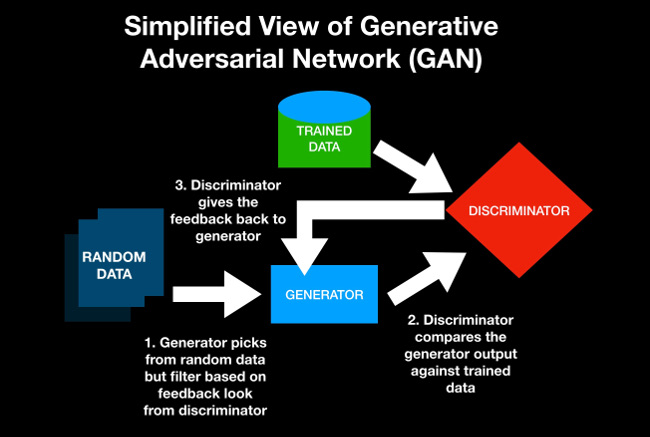GAN的实际实现要比这复杂得多，但这是一个总的想法。
理解GAN的最简单方法是考虑一个场景，其中侦探和伪造者正在玩重复性的猜谜游戏，其中伪造者试图制造100美元的钞票的伪造品，而侦探则判断每个物品是真品还是假品。 这是这种情况的假设对话：

造假者： “这100美元看起来像真钱吗？”  侦探： “不！那看上去根本不是一张真正的100美元钞票。”  伪造者 （持有一种新设计）：“这张100美元的钞票看起来像真钱吗？”  侦探： “那更好，但仍不如真实。”  伪造者 （持有设计的变体）：“现在呢？”  侦探： “那很接近，但仍然不像真实。”  伪造者 （持有另一个变种）：“这应该像真实的，对吧？”  侦探： “是的！那非常接近！”

好。 因此，侦探实际上是犯罪分子。 在这个例子中，伪造者是产生者，而侦探是鉴别者。 随着游戏的反复进行，伪造货币与真实货币相似的可能性可能会越来越高。
假冒伪劣侦探示例与GAN之间的主要区别在于GAN的主要目的不是创建一个只有一个答案的100％实际输出。 相反，您可以将GAN视为某种超级创意引擎 ，它可以生成许多意外的输出，这些输出既可以使您感到惊讶，又可以满足您的好奇心和期望。
机器学习
在开始使用GAN之前，您需要一些有关机器学习及其背后的数学知识的背景知识。
如果您不熟悉机器学习，则可以免费使用Goodfellow的Deep Learning教科书 ，也可以根据需要通过其网站上的Amazon链接购买印刷版。
但是，开始进行机器学习的最佳方法可能是通过大规模开放式在线课程（MOOC）上免费课程，例如：
既然数据科学对机器学习有所帮助，请了解Kaggle ，这是最著名的数据科学家和机器学习者在线社区
您还将希望熟悉最受欢迎的机器学习工具：
张量流  火炬
另外，请务必查看有关机器学习的其他Opensource.com文章：
当今机器学习入门  使用机器学习为卡通着色  使用机器学习为颜色命名  适用于Python的3大机器学习库  使用Python进行机器学习：基本的技巧和窍门
GAN入门
现在，我们了解了有关机器学习和GAN的一些基础知识，您可能已经准备好开始使用GAN。 首先，根据BSD 3-条款“ New”或“ Revised”许可在GitHub上访问Goodwell的原始GAN代码 。
GAN也有几种变体：
火炬实施  深度卷积生成对抗网络 （DCGANN）  周期一致的对抗网络 （CCAN）
您可以使用GAN的方式
GAN有无限可能的用例，但以下是人们尝试过的一些方法：
字体生成： zi2zi  动漫角色生成： animeGAN  交互式图像生成： iGAN  文本到图像： TAC-GAN和“ 生成对抗性文本到图像合成 ”  3D对象生成： pix2vox  图像编辑： IcGAN  视频生成： 对抗性视频生成和“ 使用预测性生成网络的无监督视觉结构学习 ”  音乐生成：“ MidiNet ：用于符号域音乐生成的卷积生成对抗网络”
您使用过GAN吗？ 您还有其他资源要分享吗？ 如果是这样，请在评论中留下注释。

生成对抗网络和对抗神经网络

展开全文python 机器学习 人工智能 深度学习
• 通过以端对端方式从LR到HR域转换的生成对抗网络（GAN）框架进行训练，我们提出了超分辨率残留循环生成对抗网络（SRResCycGAN）。 我们在定量和定性实验中证明了我们提出的方法，该方法很好地推广到了真实图像的超...
• 在ICCV 2019会上，武汉大学的一篇论文针对这一问题提出了一种用于阴影检测和去除的注意循环生成对抗网络——ARGAN。论文中通过生成一张更加准确的注意力图，用来逐渐显示检测出的阴影区域，然后还原到一张更加真实的...
• 该模型基于循环生成对抗网络结构学习无配对 SD-OCT和EDI-oCT图像之间的域映射关系。为了克服循环生成对抗网络生成图像的结构性差异问题，模型利用连续帧之间的相似性引入全局结构损失，保证了图像的全局结构一致性;...
• 针对低剂量医学CT图像噪声大且配对数据集难以获得的问题,提出一种基于改进型循环一致性生成对抗网络的低剂量CT去噪算法。该算法使用循环一致性生成对抗网络,由未配对的数据集实现了从低剂量CT图像到标准剂量CT图像的...
• 生成对抗网络 图像生成Machines are generating perfect images these days and it’s becoming more and more difficult to distinguish the machine-generated images from the originals.如今，机器正在生成完美...python java 机器学习 计算机视觉
• 该方法基于生成对抗网络，直接将源域数据映射到目标域数据中。双循环迁移排序模型主要有两部分：（1）让两个数据域互相学习对方域的特征分布信息部分，（2）使用已经嵌入目标域特征信息的源域数据来训练排序模型部分...
• GAN (生成对抗网络) 手写数字图片生成 文章目录GAN (生成对抗网络) 手写数字图片生成Discriminator NetworkGenerator Network简单版本的生成对抗网络判别器 Discriminator生成器 Generator超参数设置训练网络生成...神经网络 深度学习 GAN
• 生成对抗网络的判别器Generative models have been used in a myriad of applications across different domains — starting from quantitative finance to model and minimize tail risk to hydro-climate ...python java linux 机器学习
• 常见的五种神经网络 ①前馈神经网络 ②卷积神经网络 ③循环神经网络 ④深度信念网络(DBN) 深度信念网络（Deep Belief Netword, DBN）是一种深层的概率有向图模型，其图结构有...⑤生成对抗网络 ...
• 循环生成对抗网络（简称CycleGans）是功能强大的计算机算法，具有改善数字生态系统的潜力。 它们能够将信息从一种表示形式转换为另一种表示形式。 例如，当给定图像时，他们可以对其进行模糊处理，着色（如果...GAN 人工智能 神经网络
• ## Pytorch Note45 生成对抗网络（GAN）

多人点赞 热门讨论 2021-08-13 21:28:08
Pytorch Note45 生成对抗网络（GAN） 文章目录Pytorch Note45 生成对抗网络（GAN）GANsDiscriminator NetworkGenerator Network简单版本的生成对抗网络判别网络生成网络 全部笔记的汇总贴：Pytorch Note 快乐星球 ...GANs 生成器
• 简介 CycleGAN是在2017年发表的一篇文章《Unpaired Image-to-Image Translation using Cycle-Consistent Adversarial Networks》，同一时期还有两篇非常...传统的GAN是单向生成，而CycleGAN是互相生成网络是个环...CycleGAN
• 神经网络分很多种, 有普通的前向传播神经网络 , 有分析图片的 CNN 卷积神经网络 , 有分析序列化数据, 比如语音的 RNN 循环神经网络...生成对抗网络（GAN, Generative Adversarial Networks ）是一种深度学习模型，...
• 可大致分为三种基础模型，首先的就是卷积神经网络（Convolutional Neural Networks, CNNs，1998）、循环神经网络（RecurrentNeural Networks, RNNs，2014）、和生成对抗网络（Generative Adversarial Nets, GANs，...深度学习 机器学习人工智能 计算机视觉 机器学习
•贝叶斯
• 在ICCV 2019会上，武汉大学的一篇论文针对这一问题提出了一种用于阴影检测和去除的注意循环生成对抗网络——ARGAN。论文中通过生成一张更加准确的注意力图，用来逐渐显示检测出的阴影区域，然后还原到一张更加真实的...
• GAN简介 生成对抗网络GAN主要由两部分组成：生成网络和判别网络。每个网络都可以是任何神经网络，比如普通的人工神经网络（artificial neural network，ANN）、...生成对抗网络的基本结构图如图所示，对于生成对抗网络python 机器学习
• 深度卷积生成对抗网络（DCGAN） ---- 生成 MNIST 手写图片 1、基本原理 生成对抗网络（GAN）由2个重要的部分构成： 生成器(Generator)：通过机器生成数据（大部分情况下是图像），目的是“骗过”判别器 判别器...卷积神经网络 DCGAN 深度学习
• 1 生成对抗网络原理 GAN 的核心思想源于博弈论的纳什均衡。 设定参与游戏的双方分别为一个生成器(Generator)和一个判别器(Discriminator), 生成器捕捉真实数据样本的潜在分布, 并生成新的数据样本; 判别器是一个二...机器学习计算机视觉 深度估计
• 生成对抗网络模型(GAN)是基于深度学习的一种强大的生成模型，可以应用于计算机视觉、自然语言处理、半监督学习等重要领域。生成对抗网络最最直接的应用是数据的生成，而数据质量的好坏则是评判GAN成功与否的关键...GAN 深度学习 迁移学习 机器学习...